Extension of bars

# Uniform bars:

Consider the problem of calculating the extension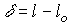due to the application of an axial load P on a uniform bar as shown in the figure.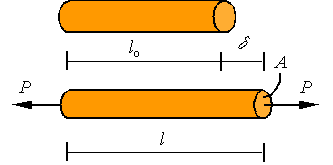A bar is considered uniform if its cross-sectional area and elastic modulus are constant along the length of the bar. For such a bar under axial loading, the stress and strain along the bar are constants and given by the expressions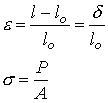Assuming small strains and substituting these expressions into Hooke’s law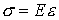, one arrives at the equation for the extension of the bar given as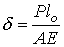# Non-uniform bars:

A bar with a varying cross-sectional area is an example of non-uniform bar. In general, one can have varying cross section, elastic modulus, and axial load in a bar. Such situations may result in varying stress and strain along the bar. This, in turn, requires a more in depth analysis to evaluate the extension of the bar. First, let us setup the kinematics of the deformation. As shown in the figure, in a typical situation one would have an initial unloaded configuration (top) and a configuration after loading (bottom).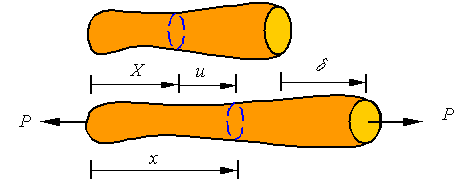A typical cross-section that is at location X in the initial configuration, ends up in location x in the current configuration by being displaced a distance u from its original location. Each cross section can be displaced a different amount and may have a different cross-sectional area. Therefore, the displacement and cross-sectional area are each a function of the location along the bar (i.e., u(X) and A(X)). A typical segment of length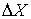of the bar is shown in the following figure.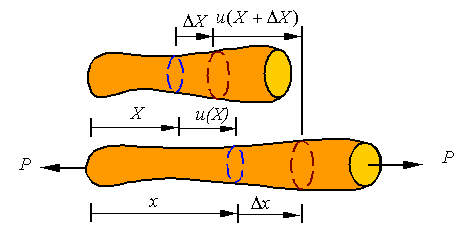The average strain in this segment of the bar is given by the expressionOne can take a limit asgoes to zero to define the strain at each point of the bar by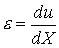The extension of any segment of the bar is the difference between the displacement of one end minus the displacement of the other end. This expression for strain can be integrated to get the extension. Let us say we are looking for the extension of the segment between X1 and X2. This would be given by integrating the strain to get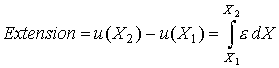The total extension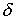of a bar of initial length L is given by the expression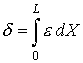As shown in the figure below, the bar might have a distributed load w applied on it in addition to point loads. As a result, the load and cross-sectional area might both vary with location in the bar so we will denote the load in the section at X by P(X) and the cross-sectional area by A(X). Therefore, the axial stress is given by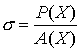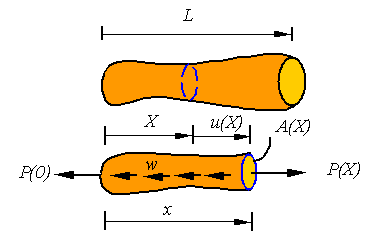Since the material can change along the bar, the elastic modulus may also be a function of location along the bar. From Hooke’s law it follows that the strain at each point of the bar is given by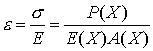Therefore the total extension in a non-uniform bar is given by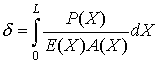This expression reduces to the one for a uniform bar when the argument of the integral is constant.